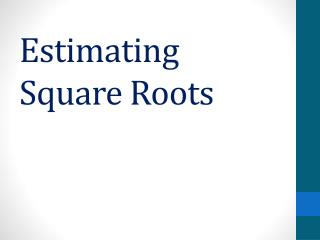# Estimating Square Roots - PowerPoint PPT PresentationDownload PresentationEstimating Square Roots

Estimating Square RootsDownload Presentation## Estimating Square Roots

- - - - - - - - - - - - - - - - - - - - - - - - - - - E N D - - - - - - - - - - - - - - - - - - - - - - - - - - -
##### Presentation Transcript

1. Estimating Square Roots

2. In order to estimate square roots you must first know your perfect squares. • A perfect square is the number you get when you multiply a number by itself.

3. For instance • 1 is a perfect square because 1X1=1 • 4 is a perfect square because 2x2=4. • 9 is a perfect square because 3x3=9.

4. List all the perfect squares from 1 to 400. • 1 4 9 16 • 25 36 49 64 • 81 100 121 169 • 196 225 256 289 • 324 361 400

5. Now find the perfect squares for 25, 30, 40, and 50. • 625 • 900 • 1600 • 2500

6. Now your ready to estimate square roots. Estimate between which two whole numbers the following square roots will fall on a number line. • 2. 3. • 5. 6.